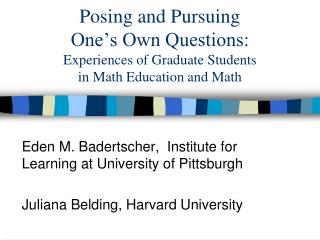# Posing and Pursuing One’s Own Questions: Experiences of Graduate Students in Math Education and Math - PowerPoint PPT PresentationDownload PresentationPosing and Pursuing One’s Own Questions: Experiences of Graduate Students in Math Education and Math

Posing and Pursuing One’s Own Questions: Experiences of Graduate Students in Math Education and MathDownload Presentation## Posing and Pursuing One’s Own Questions: Experiences of Graduate Students in Math Education and Math

- - - - - - - - - - - - - - - - - - - - - - - - - - - E N D - - - - - - - - - - - - - - - - - - - - - - - - - - -
##### Presentation Transcript

1. Posing and Pursuing One’s Own Questions:Experiences of Graduate Students in Math Education and Math Eden M. Badertscher, Institute for Learning at University of Pittsburgh Juliana Belding, Harvard University

2. Overview of the Course • Main Goal: Students develop ability and desire to ask and investigate their own mathematical questions • Other Goals: Mathematical communication, Appreciation for process/struggle of math • Who: Graduate students in Math Ed (also Physics Ed and Mathematics)

3. Structure of Course • In-Class Investigations • Weekly 2 hr. classes • Three topics (last 4-5 weeks each) • Small groups, informal presentations • Individual Projects • Student-designed, Outside of class • Studio Times (1 hr., 5 during semester) • Final written project • Journals (in-class and weekly project updates)

4. In-Class Investigations: Wrestling with… • 1: Rational Numbers Farey Sequences, Representation of Rationals • 2: Geometry Definitions of A Parabola, Taxi-cab Geometry • 3: The Real Numbers Cardinality, Representation of Reals

5. The Creative Process in Mathematics “Mathematics has two faces. Presented in finished form, mathematics appears as a purely demonstrative [deductive] science, but mathematics in the making is a sort of experimental science. A correctly written mathematical paper is supposed to contain strict demonstrations only, but the creative work of the mathematician resembles the creative work of the naturalist; observation, analogy, and conjectural generalizations, or mere guesses play an essential role in both.” -Polya, 1952

6. Mathematical Themes Through the creative process, students develop need for and appreciation of Definitions (their role, principled choices, sense-making) What constitutes a proof? Multiple viewpoints (geometric, algebraic, etc.) Precise mathematical language and notation

7. “What-If-Not” From The Art of Problem Posing, Brown and Walter, 1983 Given a mathematical object/situation/problem: • List attributes • Ask “what-if-not” (tweak attributes) • Formulate new questions

8. Example: A Parabola • Definition: The locus of points equidistant from a line (directrix) and a point not on the line (focus) • What-if-not… • Not Equidistant (1/2 as far, 2x as far) • Directrix is another object (circle, a parabola) • Focus is on the line (degenerate conics) • Non-Euclidean distance (Taxi-cab geometry)

9. Other Resources • Habits of Mind: An Organizing Principle of Mathematics Curricula, Cuoco, Goldenberg and Mark. 1996 • The Roles of The Aesthetic in Mathematical Inquiry, Sinclair, 2004

10. Instructors’ Role • In class: • participant (“having new eyes”) • translator (model communication) • facilitator (restart, regroup and recap) • Outside of class: • reframe questions • restructure groups according to interest, facility with formal math, working style and new questions that arise…

11. The Students’ Experience “It made me understand what it means to explore math as opposed to learn math and solve problems” (from student’s reflection) • Freedom of exploration/“Playfulness” • Increased confidence in math & validity of own questions • Improved communication of mathematics (through journals/project write-ups)

12. What can a Mathematician (in training) gain? • Challenges: • Being the know-it-all “math guy” • When to hold back, when to contribute • Some topics already familiar • At times, less rigorous than used to

13. What can a Mathematician (in training) gain? • Benefits: • More freedom to ask questions, make guesses (than in graduate math classes) • Exposure to more experimental, intuitive, “naïve” approaches to math • Practice for graduate research (formulating a thesis question, trying multiple approaches)

14. Why? All learners (educators in particular) need hands-on experience with.. • how math is created (what do mathematicians do?) • how to communicate about math across disciplines (eg: Hy Bass) • how to learn math outside a classroom or textbook

15. For More Information • CSSM Institute at Educational Development Center, Newton, MA http://cssm.edc.org/AboutCSSM.html • Learning to learn Mathematics: Voices of doctoral students in mathematics education. In M. Strutchens & W. Gary Martin (eds.) The Learning of Mathematics. 69th Yearbook of the National Council of Teachers of Mathematics. NCTM: Reston, VA.

16. Appendix: Getting Into the Problems • How did we set up each of the investigations?

17. Rationals

18. Geometry For each definition, locus of points equidistant from focus and directrix locus made from cutting plane parallel to side of cone equation of form y = ax^2 + bx + c Generate attributes, what-if-nots and questions.